• 修炼Pandas 生成DataFrame、读取和保存数据


文章目录

一、生成对象
二、读取数据
三、保存数据

一、生成对象
以字典形式生成
import pandas as pd

datas = {

'排名': [1, 2, 3, 4, 5],

展开全文python 数据分析
• 生成dataframe的两种方式分别为：使用data，index，column方法和使用字典输入的方法，见代码： import pandas as pd column_1 = [1,2,3] column_2 = ['hunan','hubei','beijing'] column_3 = [[1,'hubai'],[2,'...
生成dataframe的两种方式分别为：使用data，index，column方法和使用字典输入的方法，见代码：
import pandas as pd
column_1 = [1,2,3]
column_2 = ['hunan','hubei','beijing']

column_3 = [[1,'hubai'],[2,'hunan'],[3,'beijing']]

df = pd.DataFrame({'省份' : column_2, '人数' : column_1})
df1 = pd.DataFrame(data = column_3,index = [1,2,3],columns=['人数','省份'])

df,df1的结果都为：展开全文python
• 一、创建dataframe C:\Windows\system32>python Python 3.5.4 (v3.5.4:3f56838, Aug 8 2017, 02:17:05) [MSC v.1900 64 bit (AMD64)] on win32 Type "help", "copyright", "credits" or "license" for m...
lib不用就忘：
一、创建dataframe
C:\Windows\system32>python
Python 3.5.4 (v3.5.4:3f56838, Aug  8 2017, 02:17:05) [MSC v.1900 64 bit (AMD64)] on win32
>>> import pandas as pd
>>> a = [[3, 4], [5, 6],[7,8]]
>>> b = pd.DataFrame(a)
>>> b
0  1
0  3  4
1  5  6
2  7  8
二、索引
# dataframe的行索引为index，列索引为columns

# 改变索引，会改变dataframe本身，如果不想改变dataframe本身，最好先复制再修改。
new_index1 = ['d', 'e', 'f']
b.index=new_index1
0  1
d  3  4
e  5  6
f  7  8

# 改变列索引
new_index2 = ['g', 'h']
b.columns=new_index2
g  h
0  3  4
1  5  6
2  7  8
三、获取行或列
>>> b
0  1
d  3  4
e  5  6
f  7  8

# 三种索引方式：loc, iloc, ix
# loc通过行或列的标签索引，iloc通过行号或列号索引，ix是前两种方式的综合

# 获取第一行
# 方式一：通过loc获取行或列标签的方式
>>> b.loc[['e'], :] # 获取行标签为'e'的行，也可以用 b.loc['e', :]

# 输出：
0  1
e  5  6

# 方式二：通过iloc获取行号或者列号的方式
>>> b.iloc[:, 1] # 获取列标签为1的列，也可以用 b.iloc[:,]

# 输出：
d    4
e    6
f    8

# 方式三：通过ix灵活获取。行列标签和行号列号都支持
>>> b.ix['e',:]

# 输出：
__main__:1: FutureWarning:
.loc for label based indexing or
.iloc for positional indexing

See the documentation here:
http://pandas.pydata.org/pandas-docs/stable/user_guide/indexing.html#ix-indexer-is-deprecated
0    5
1    6
Name: e, dtype: int64

>>> b.ix[:,1]

# 输出：
d    4
e    6
f    8
Name: 1, dtype: int64
四、获取出的行或列的类型就是dataseries
>>> type(b.loc['e', :])
<class 'pandas.core.series.Series'>
>>> type(b.iloc[:,1])
<class 'pandas.core.series.Series'>

展开全文• 文章目录1.pandas.DataFrame.query官方案例2.pandas.DataFrame.reset_index官方案例将reset_index与MultiIndex一起使用 1.pandas.DataFrame.query 官网 DataFrame.query(expr, inplace=False, **kwargs) 描述 使用...


文章目录
1.pandas.DataFrame.query官方案例
2.pandas.DataFrame.reset_index官方案例将reset_index与MultiIndex一起使用

1.pandas.DataFrame.query
官网
DataFrame.query(expr, inplace=False, **kwargs)

描述

使用布尔表达式查询DataFrame的列

参数

expr : str 要计算的查询字符串

inplace : bool 查询是应该修改数据还是返回修改后的副本

**kwargs

返回

DataFrame or None 由提供的查询表达式生成的DataFrame；如果inplace = True，则为None

官方案例
df = pd.DataFrame({'A': range(1, 6),
'B': range(10, 0, -2),
'C C': range(10, 5, -1)})

df
A   B  C C
0  1  10   10
1  2   8    9
2  3   6    8
3  4   4    7
4  5   2    6

df.query('A > B')
A  B  C C
4  5  2    6

# 前面的表达式等价于
df[df.A > df.B]
A  B  C C
4  5  2    6

# 对于名称中有空格的列，可以使用反引号
df.query('B == C C')
A   B  C C
0  1  10   10

# 前面的表达式等价于
df.query('B == C C')
A   B  C C
0  1  10   10

2.pandas.DataFrame.reset_index
官网
DataFrame.reset_index(level=None, drop=False, inplace=False, col_level=0, col_fill='')

描述
重置索引或索引的级别。
重置DataFrame的索引，并使用默认索引。
如果DataFrame具有MultiIndex，则此方法可以删除一个或多个级别

参数

level : int, str, tuple, or list, default None 仅从索引中删除给定的级别,默认情况下删除所有级别。

drop : bool, default False 不要尝试将索引插入dataframe columns,这会将索引重置为默认的整数索引

inplace : bool, default False if True,则在原对象上修改 if False, 则返回修改后的对象

col_level : int or str, default 0 如果列有多个级别，请确定将标签插入到哪个级别。默认情况下，它被插入第一级。

col_fill : object, default ‘’ 如果列具有多个级别，请确定如何命名其他级别。如果为None，则重复索引名称。

返回

DataFrame or None if False,具有新索引的DataFrame；如果inplace = True，则为None

官方案例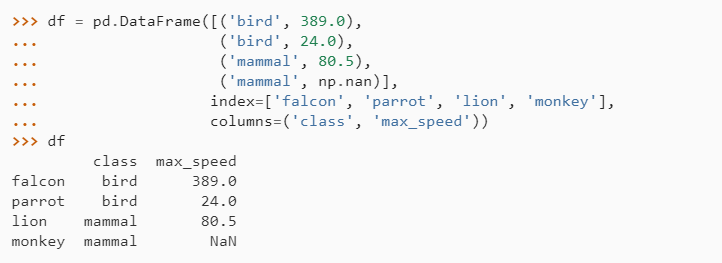重置索引时，会将旧索引添加为列，并使用新的顺序索引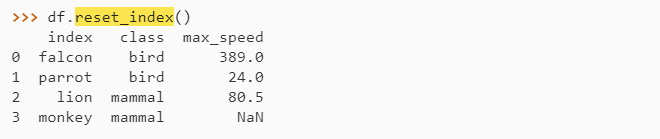我们可以使用drop参数来避免将旧索引添加为列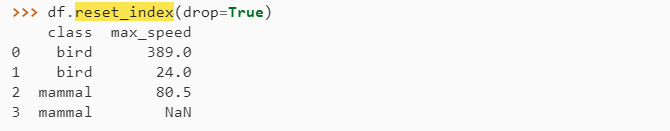将reset_index与MultiIndex一起使用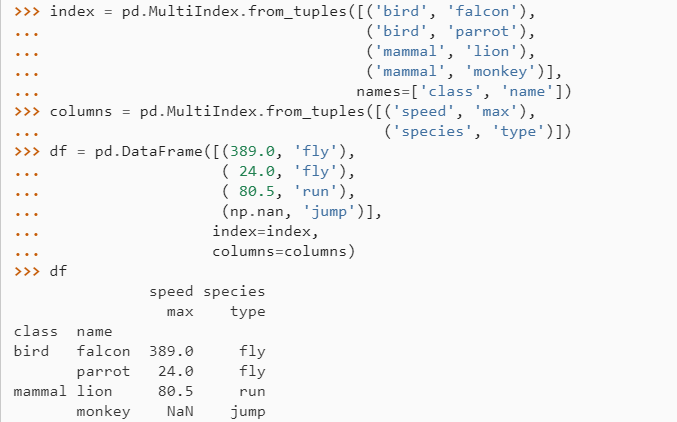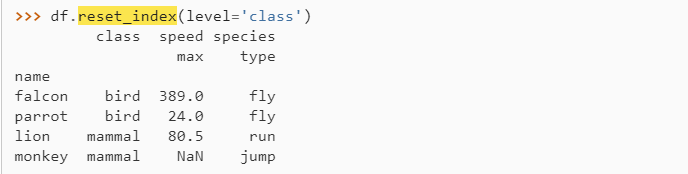如果我们不删除索引，则默认情况下，它位于顶层。 我们可以将其放在另一个级别：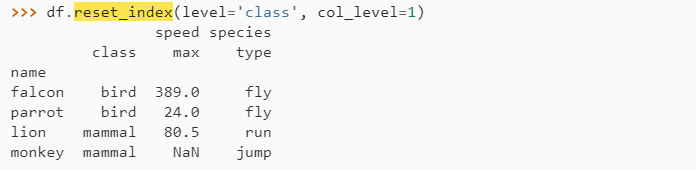当索引插入到另一个级别下时，我们可以使用参数col_fill指定哪个级别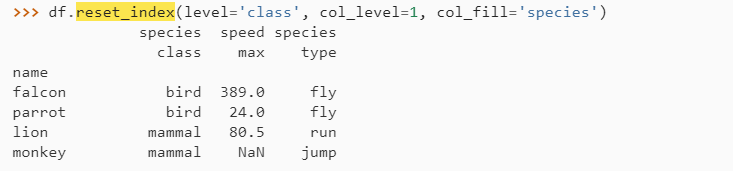如果我们为col_fill指定了不存在的级别，则会创建它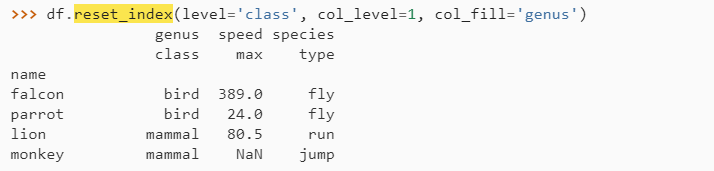展开全文query
• pandas提取DataFrame数据集中某几列python 大数据
• pandas生成DataFrame时，Series为数值类型则会把空值视为NaN。而spark在处理NaN时并不视为Null，有可能会导致问题。例如： >>> pdf = pd.DataFrame([(0,‘Alice’,1.1),(1,‘Bob’,None),(2,None,3.3)], ...spark
• 主要介绍了Python中pandas模块DataFrame创建方法,结合实例形式分析了DataFrame的功能,以及pandas模块基于列表、字段与数组创建DataFrame的相关操作技巧,需要的朋友可以参考下
• 众所周知，pandas生成透视表后，index会变成multiindex，即多层索引。 多层索引就是列索引大于一层的情况。 实际操作中透视表生成之后想要与其他一层索引的表格进行merge而因为出现多层索引所以无法实现正常的...multiindex pivot
• 创建pandasDataFrame对象的5种方法 PandasDataFrame对象可以通过许多方式创建，举几个常用的例子。 通过Series对象创建 #1、通过单个Series对象创建。DataFrame是一组Series对象的集合，可以用单个Series创建...
• pandas.DataFrame 基本技巧head / tail / .T：数据查看、转置del / drop：删除对齐排序.sort_values：按值排序.sort_index：索引排序 head / tail / .T：数据查看、转置 import numpy import pandas df = pandas....python numpy 数据分析
• 31_Pandas.DataFrame，Series和...从NumPy数组ndarray生成DataFrame 有关内存共享的注意事项（查看和复制） Pandas0.24.0或更高版本：to_numpy（） 每个示例都将用示例代码进行描述。 此外，pandas.DataFrame和pandapython 机器学习
• 贾丁 jardin （名词，法语） –花园，院子，小树林... Jardin是适用于Python应用程序的基于pandas.DataFrame的ORM。 最新的Pypi版本 生成状态（Travis） 安装 \$ pip install jardin 文献资料 查看完整的文档和教程。
• 本文介绍的是python中pandas.DataFrame对行与...生成DataFrame数据 df = DataFrame(np.random.randn(4, 5), columns=['A', 'B', 'C', 'D', 'E']) DataFrame数据预览： A B C D E 0 0.673092 0.230338 -0.171681 0.3
• df = DataFrame(columns=('lib', 'qty1', 'qty2'))#生成空的pandas表 for i in range(5):#插入一行<span id=transmark xss=removed></span> df.loc[i] = [randint(-1,1) for n in range(3)] print df 以上这篇...
• 下面小编就为大家带来一篇对pandasdataframe绘图并保存的实现方法。小编觉得挺不错的，现在就分享给大家，也给大家做个参考。一起跟随小编过来看看吧
• pandas.DataFrame对行和列求和及添加新行和列 pandas.DataFrame对行和列求和及添加新行和列 导入模块： from pandas import DataFrame ...生成DataFrame数据 df = DataFrame(np.random.ran...
• Python之pandasDataFrame import pandas as pd import math df=pd.DataFrame({'key':['a','b','c'],'data1':[1,2,3]}) 1、生成新列 df['newcol'] = df['data1'].apply(lambda x: math.sin(x)...python
• 14_Pandas.DataFrame行和列的转置 如果要交换（转置）pandas.DataFrame的行和列，使用T属性或transpose（）方法。 这两种方法都不会保留原始对象不变，也不会返回交换了行和列（转置）的新对象。请注意，根据每一列...python 机器学习
• 主要介绍了从列表或字典创建PandasDataFrame对象的方法，文中通过示例代码介绍的非常详细，对大家的学习或者工作具有一定的参考学习价值，需要的朋友们下面随着小编来一起学习学习吧
• import pandas as pd import csv import random # 下面一段代码生成一个用来模拟统计的表格 # simulation = pd.DataFrame(columns=('user_id', 'industry', 'category', 'click_times')) # for i in range(100): # ...python
• 33_Pandas.DataFrame，Series和Python标准列表的相互转换 pandas.DataFramepandas.Series和Python标准list类型列表可以相互转换。 这里，将描述以下内容。 将list类型列表转换为pandas.DataFramepandas.Series ...python 机器学习
• DataFrame2.1 DataFrame结构2.2 DataFrame属性2.3 DatatFrame索引的设置2.3.1 修改行列索引值2.3.2 重设索引2.3.3 以某列值设置为新的索引 1. Pandas介绍 专门用于数据挖掘的开源python库 便捷的数据处理能力 ...自然语言处理 数据挖掘 机器学习
• 本文详细讲解python pandas.dataframe的常见操作大全python 经验分享 程序人生
• 下面小编就为大家分享一篇pandas.DataFrame 根据条件新建列并赋值的方法，具有很好的参考价值，希望对大家有所帮助。一起跟随小编过来看看吧
• ## pandas之DataFrame绘图

万次阅读 多人点赞 2018-06-19 18:25:34
我们知道Pandas库中有两种数据结构一种是Series结构类型的数据，还有一个种就是DataFrame类型的数据，那么今天我们就来聊一聊DataFrame结构类型的数据绘图。 我们先来看一个最简单的例子。试试我们的小心脏会不会......